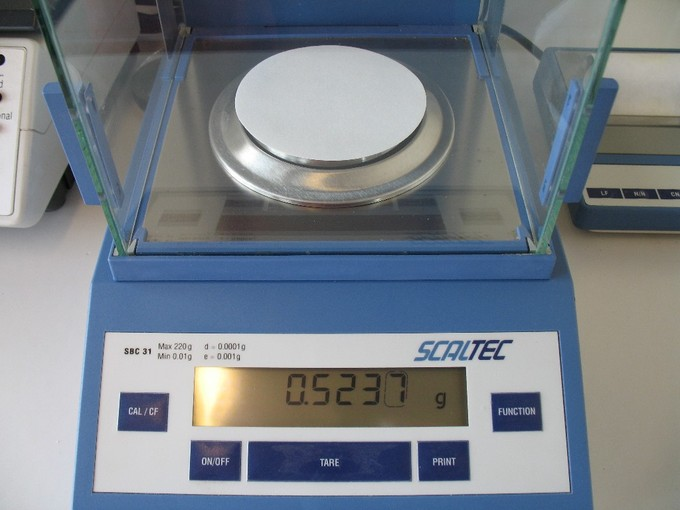## Exact Numbers

#### Learning Objective

• Recognize exact and measured numbers

#### Key Points

• An exact number has absolutely no uncertainty in it.
• Exact numbers cannot be simplified and have an infinite number of significant figures.
• Measured numbers have a limited number of significant figures.

#### Term

• Exact numbersThese numbers are either defined numbers or result of a count. They have an infinite number of significant figures.

## Exact Numbers

Exact numbers are either defined numbers or the result of a count. For example, a dozen is defined as 12 objects, and a pound is defined as 16 ounces. An exact number can only be expressed in one way and cannot be simplified any further. Exact numbers have an infinite number of significant figures, but they often appear as integers.Exact numbersThere are exactly two chairs in this picture. The number of chairs is counted, not measured, so we are completely certain how many chairs there are.

Examples of exact numbers include:

• Conversions within the American system (such as pounds to ounces, the number of feet in a mile, the number of inches in a foot, etc).
• Conversions with the metric system (such as kilograms to grams, the number of meters in a kilometer, the number of centimeters in a meter).
• The words per and percent mean exactly out of one or one hundred, respectively.
• Counted numbers are exact: there are two chairs in the photograph. There are fifteen books on the shelf. Eighty-seven people attended the lecture.

## Measured Numbers

In contrast, measured numbers always have a limited number of significant digits. A mass reported as 0.5 grams is implied to be known to the nearest tenth of a gram and not to the hundredth of a gram.

There is a degree of uncertainty any time you measure something. For example, the weight of a particular sample is 0.825 g, but it may actually be 0.828 g or 0.821 g because there is inherent uncertainty involved. On the other hand, because exact numbers are not measured, they have no uncertainty and an infinite numbers of significant figures.Measured numbersMass is an example of a measured number. When mass is reported as 0.5237 g, as shown on this scale, it is more precise than a mass reported as 0.5 g.

Examples of measured numbers:

• The diameter of a coin, such as 10.2 mm.
• The weight of an object, such as 8.887 grams.
• The length of a pen, such as 12 cm.